# MySQL(基础篇)之MySQL函数（字符串函数）分析

java哥 阅读：288 2021-03-31 23:15:16 评论：0

`ASCII(str)``ORD(str)`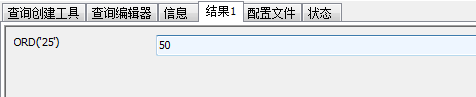`CONV(N,from_base,to_base)`

```mysql> select CONV("a",16,2);
-> '1010'
mysql> select CONV("6E",18,8);
-> '172'
mysql> select CONV(-17,10,-18);
-> '-H'
mysql> select CONV(10+"10"+'10'+0xa,10,10);
-> '40'```
```

`BIN(N)`

返回二进制值

`N`的一个字符串表示，在此

`N`是一个长整数(

`BIGINT`) 数字，这等价于

`CONV(N,10,2)`。如果

`N`是

`NULL`，返回

`NULL`。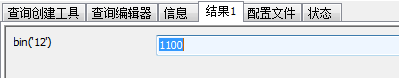```
`OCT(N)`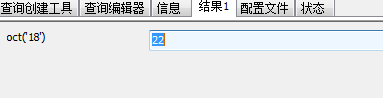`HEX(N)`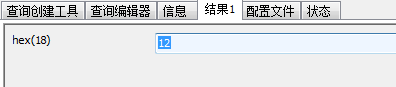`CHAR(N,...)`
`CHAR()`将参数解释为整数并且返回 由这些整数的ASCII代码字符组成的一个字符串。 `NULL`值 被跳过。
```mysql> select CHAR(77,121,83,81,'76');
-> 'MySQL'
mysql> select CHAR(77,77.3,'77.3');
-> 'MMM'```
```

`CONCAT(str1,str2,...)`

返回来自于参数连结的字符串。如果任何参数是

`NULL`， 返回

`NULL`。可以有超过2个的参数。一个数字参数被变换为等价的字符串形 式。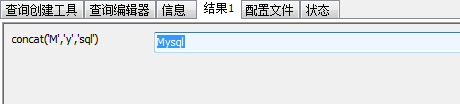```
`LENGTH(str)`

`OCTET_LENGTH(str)`

`CHAR_LENGTH(str)`

`CHARACTER_LENGTH(str)`              返回字符串 `str`的长度。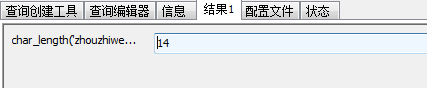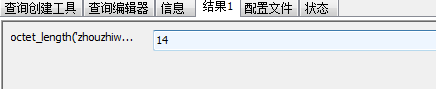注意，对于多字节字符，其CHAR_LENGTH()仅计算一次。

`LOCATE(substr,str)`

`POSITION(substr IN str)`

```mysql> select LOCATE('bar', 'foobarbar');
-> 4
mysql> select LOCATE('xbar', 'foobar');
-> 0
```
`该函数是多字节可靠的。  `
`LOCATE(substr,str,pos)`

```mysql> select LOCATE('bar', 'foobarbar',5);
-> 7
```

`INSTR(str,substr)`

```mysql> select INSTR('foobarbar', 'bar');
-> 4
mysql> select INSTR('xbar', 'foobar');
-> 0
```

`LPAD(str,len,padstr)`

```mysql> select LPAD('hi',4,'??');
-> '??hi'
```
`RPAD(str,len,padstr)`

```mysql> select RPAD('hi',5,'?');
-> 'hi???'
```
`LEFT(str,len)`

```mysql> select LEFT('foobarbar', 5);
-> 'fooba'
```

`RIGHT(str,len)`

```mysql> select RIGHT('foobarbar', 4);
-> 'rbar'
```

`SUBSTRING(str,pos,len)`

`SUBSTRING(str FROM pos FOR len)`

`MID(str,pos,len)`

```mysql> select SUBSTRING('Quadratically',5,6);
-> 'ratica'
```

`SUBSTRING(str,pos)`

`SUBSTRING(str FROM pos)`

```mysql> select SUBSTRING('Quadratically',5);
-> 'ratically'
mysql> select SUBSTRING('foobarbar' FROM 4);
-> 'barbar'
```

`SUBSTRING_INDEX(str,delim,count)`

```mysql> select SUBSTRING_INDEX('www.mysql.com', '.', 2);
-> 'www.mysql'
mysql> select SUBSTRING_INDEX('www.mysql.com', '.', -2);
-> 'mysql.com'
```

`LTRIM(str)`

```mysql> select LTRIM('  barbar');
-> 'barbar'
```
`RTRIM(str)`

```mysql> select RTRIM('barbar   ');
-> 'barbar'
```
`该函数对多字节是可靠的。  `
`TRIM([[BOTH | LEADING | TRAILING] [remstr] FROM] str)`

```mysql> select TRIM('  bar   ');
-> 'bar'
mysql> select TRIM(LEADING 'x' FROM 'xxxbarxxx');
-> 'barxxx'
mysql> select TRIM(BOTH 'x' FROM 'xxxbarxxx');
-> 'bar'
mysql> select TRIM(TRAILING 'xyz' FROM 'barxxyz');
-> 'barx'
```

`SOUNDEX(str)`

```mysql> select SOUNDEX('Hello');
-> 'H400'
-> 'Q36324'
```
`SPACE(N)`

```mysql> select SPACE(6);
-> '      '
```
`REPLACE(str,from_str,to_str)`

```mysql> select REPLACE('www.mysql.com', 'w', 'Ww');
-> 'WwWwWw.mysql.com'
```

`REPEAT(str,count)`

```mysql> select REPEAT('MySQL', 3);
-> 'MySQLMySQLMySQL'
```
`REVERSE(str)`

```mysql> select REVERSE('abc');
-> 'cba'
```

`INSERT(str,pos,len,newstr)`

```mysql> select INSERT('Quadratic', 3, 4, 'What');
-> 'QuWhattic'
```

`ELT(N,str1,str2,str3,...)`

```mysql> select ELT(1, 'ej', 'Heja', 'hej', 'foo');
-> 'ej'
mysql> select ELT(4, 'ej', 'Heja', 'hej', 'foo');
-> 'foo'
```
`FIELD(str,str1,str2,str3,...)`

```mysql> select FIELD('ej', 'Hej', 'ej', 'Heja', 'hej', 'foo');
-> 2
mysql> select FIELD('fo', 'Hej', 'ej', 'Heja', 'hej', 'foo');
-> 0
```
`FIND_IN_SET(str,strlist)`

```mysql> SELECT FIND_IN_SET('b','a,b,c,d');
-> 2
```
`MAKE_SET(bits,str1,str2,...)`

```mysql> SELECT MAKE_SET(1,'a','b','c');
-> 'a'
mysql> SELECT MAKE_SET(1 | 4,'hello','nice','world');
-> 'hello,world'
mysql> SELECT MAKE_SET(0,'a','b','c');
-> ''
```
`EXPORT_SET(bits,on,off,[separator,[number_of_bits]])`

```mysql> select EXPORT_SET(5,'Y','N',',',4)
-> Y,N,Y,N
```
`LCASE(str)`

`LOWER(str)`

```mysql> select LCASE('QUADRATICALLY');
```
`UCASE(str)`

`UPPER(str)`

```mysql> select UCASE('Hej');
-> 'HEJ'
```

`LOAD_FILE(file_name)`

```mysql> UPDATE table_name
WHERE id=1;

```

MySQL必要时自动变换数字为字符串，并且反过来也如此：

```mysql> SELECT 1+"1";
-> 2
mysql> SELECT CONCAT(2,' test');
-> '2 test'```
﻿﻿

1.本站遵循行业规范，任何转载的稿件都会明确标注作者和来源；2.本站的原创文章，请转载时务必注明文章作者和来源，不尊重原创的行为我们将追究责任；3.作者投稿可能会经我们编辑修改或补充。